RS Aggarwal Solutions: Coordinate Geometry- 1

# RS Aggarwal Solutions: Coordinate Geometry- 1 - RS Aggarwal Solutions for Class 10 Mathematics

## Exercise: 16a

Q.1. Find the distance between the points:
A(9, 3) and B(15, 11)

In this question, we have to use the distance formula to find the distance between two points which is given by, say for points P(x1,x2) and Q(y1,y2) then
PQ = √(x2 – x1)2 + (y2 – y1)2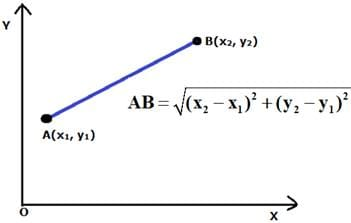AB = √{(15 – 9)2 + (11 – 3)2}
= √{(6)2 + (8)2}
= √{36 + 64}
= √100
∴ AB = 10 units.

Q.2. Find the distance between the points:
A(7, – 4) and B(– 5, 1)

In this question, we have to use the distance formula to find the distance between two points which is given by, say for points P(x1,x2) and Q(y1,y2) then
PQ = √(x2 – x1)2 + (y2 – y1)2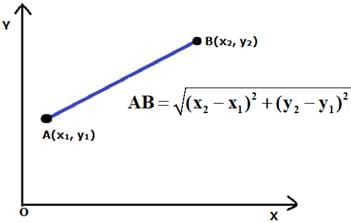AB = √{(– 5 – 7)2 + (1 – (– 4))2}
= √{(– 12)2 + (5)2}
= √{144 + 25}
= √169
∴ AB = 13 units

Q.3. Find the distance between the points:
A(– 6, – 4) and B(9, – 12)

In this question, we have to use the distance formula to find the distance between two points which is given by, say for points P(x1,x2) and Q(y1,y2) then
PQ = √(x2 – x1)2 + (y2 – y1)2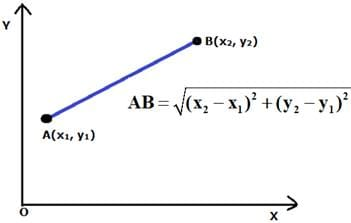AB = √{(9 – (– 6))2 + (– 12 – (– 4))2}
= √{(15)2 + (– 8)2}
= √{225 + 64}
= √289
∴ AB = 17 units

Q.4. Find the distance between the points:
A(1, – 3) and B(4, – 6)

In this question, we have to use the distance formula to find the distance between two points which is given by, say for points P(x1,x2) and Q(y1,y2) then
PQ = √(x2 – x1)2 + (y2 – y1)2AB = √{(4 – 1)2 + (– 6 – (– 3))2}
AB = √{(4 – 1)2 + (– 6 – (– 3))2}
= √{9 + 9}
= √18
∴ AB = 3√2 units

Q.5. Find the distance between the points:
P(a + b , a – b) and Q(a – b, a + b)

In this question, we have to use the distance formula to find the distance between two points which is given by, say for points P(x1,x2) and Q(y1,y2) then
PQ = √(x2 – x1)2 + (y2 – y1)2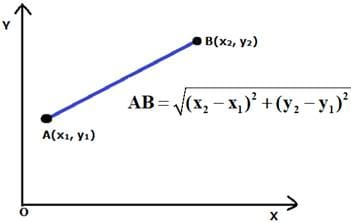AB = √{((a – b) – (a + b))2 + ((a + b) – (a – b))2}
= √{(– 2b)+ (2b)2}
= √{4b+ 4b2}
= √8b2
∴ AB = 2√2b units

Q.6. Find the distance between the points:
P(a sin a, acos a) and Q(a cos a, – a sin a)

In this question, we have to use the distance formula to find the distance between two points which is given by, say for points P(x1,x2) and Q(y1,y2) then
PQ = √(x2 – x1)2 + (y2 – y1)2PQ = √{(a cos a – a sin a)2 – (– a sin a – a cos a)2}
= √{(a2 cos2 a + a2 sin2 a – 2a2 sina.cosa + a2 cos2 a + a2 sin2 a + 2a2 sina.cosa }
= √{ a2 (cos2 a + sin2 a) + (a2 (cos2 a + sin2 a))}
= √a2(1) + a2 (1)
= √a2(1 + 1)
∴ PQ = a√2 units

Q.7. Find the distance of each of the following points from the origin:
A(5, – 12)

Since it is given that the distance is to be found from origin so in this question we have to use the distance formula keeping one – point fix i.e. O (0,0), as shown below: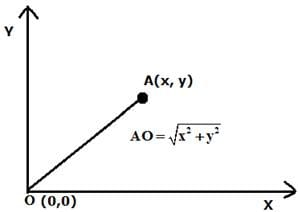OA = √{(5 – 0)2 + (– 12 – 0)2}
= √{(5)2 + (– 12)2}
= √{25 + 144}
= √169
∴ OA = 13 units

Q.8. Find the distance of each of the following points from the origin:
B(– 5, 5)

Since it is given that the distance is to be found from origin so in this question we have to use the distance formula keeping one – point fix i.e. O (0,0), as shown below:OB = √{(– 5 – 0)2 + (5 – 0)2}
= √{(– 5)2 + (5)2}
= √{25 + 25}
= √50
∴ OB = 5√2 units

Q.9. Find the distance of each of the following points from the origin:
C(– 4, – 6).

Since it is given that the distance is to be found from origin so in this question we have to use the distance formula keeping one – point fix i.e. O (0,0), as shown below: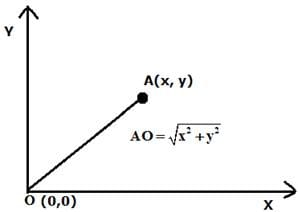OC = √{(– 4 – 0)2 + (– 6 – 0)2}
= √{(– 4)2 + (– 6)2}
= √{16 + 36}
∴ OC = √52 units

Q.10. Find all possible values of × for which the distance between the points A(x, – 1) and B(5, 3) is 5 units.

Given:
Distance AB = 5 units
By distance formula, as shown below: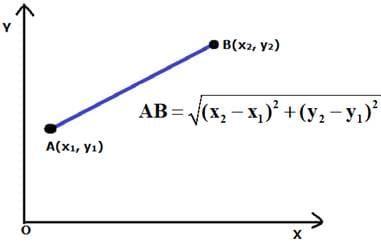AB = √{(5 – x)2 + (3 – (– 1))2}
5 = √{(5 – x)2 + (4)2}
5 = √{25 + x2 – 10x + 16}
5 = √{41 + x2 – 10x}
Squaring both sides we get
25 = 41 + x2 – 10x
⇒ 16 + x2 – 10x = 0
⇒ (x – 8)(x – 2) = 0
⇒ × = 8 or × = 2
∴ The values of × can be 8 or 2

Q.11. Find all possible values of y for which the distance between the points A(2, – 3) and B(10, y) is 10 units.

Given, the distance AB = 10 units
By distance formula, as shown below: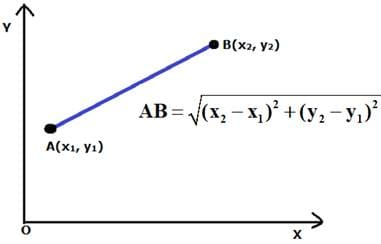AB = √{(10 – 2)2 + (y – (– 3))2}
10 = √{(8)2 + (y + 3)2}
10 = √{64 + y2 + 6y + 9}
10 = √{73 + y2 + 6y}
Squaring both sides we get
100 = 73 + y2 + 6y
On solving the equation, 100 = 73 + y2 + 6y
⇒ 27 + y2 + 6y = 0
⇒ y2 + 6y + 27 = 0
⇒ (y – 3)(y + 9) = 0
⇒ y = 3 or y = – 9
∴ The values of y can be 3 or – 9

Q.12. Find the values of x for which the distance between the points P(x, 4) and Q(9, 10) is 10 units.

Given the distance PQ = 10 units
By distance formula, as shown below: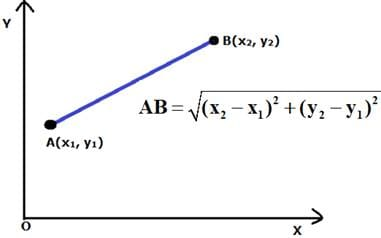PQ = √{(9 – x)2 + (10 – 4)2}
10 = √{(9 – x)2 + (6)2}
10 = √{81 + x2 – 18x + 36}
10 = √{117 + x2 – 18x}
Squaring both sides we get
⇒ 100 = 117 + x2 – 18x
⇒ x2 – 18x + 17x = 0
⇒ (x – 1)(x – 17)
⇒ × = 1 or × = 17

Q.13. If the point A(x, 2) is equidistant from the points B(8, – 2) and C(2, – 2), find the value of x. Also, find the length of AB.

Given that point A is equidistant from points B and C , so AB = AC
By distance formula, as shown below: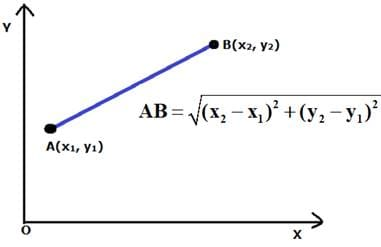AB = √{(8 – x)2 + (– 2 – 2)2}
= √{(8 – x)2 + (– 4)2}
= √{64 + x2 – 16x + 16}
= √{80 + x2 – 16x}
AC = √{(2 – x)+ (– 2 – 2)2}
= √{(2 – x)2 + (4)2}
= √{4 + x2 – 4x + 16}
= √{20 + x2 – 4x}
Now, AB = AC
Squaring both sides, we get,
(80 + x2 – 16x) = (20 + x2 – 4x)
60 = 12x
x = 5
⇒ AB = √{80 + x2 – 16x}
⇒ AB = √(80 + 52 – 16× 5)
= 5 units

Q.14. If the point A(0, 2) is equidistant from the points B(3, p) and C(p, 5), find the value of p. Also, find the length of AB.

Given that point A is equidistant from points B and C, so AB = AC
By distance formula, as shown below:AB = √{(3 – 0)2 + (p – 2)2}
= √{(3)2 + (p – 2)2}
= √{9 + p2 – 4p + 4}
⇒ AB = √{13 + p2 – 4p}
AC = √{(p – 0)2 + (5 – 2)2}
= √{(p)2 + (3)2}
⇒ AB = √{9 + p2}
Now, AB = AC
Squaring both sides, we get,
(13 + p2 – 4p) = (9 + p2)
⇒ 4 = 4p
⇒ p = 1
Now, AB = √{13 + p2 – 4p}
⇒ AB = √(13 + 1 – 4)
= √10 units
Therefore, the distance of AB = √10 units.

Q.15. Find the point on the x – axis which is equidistant from the points (2, – 5) and (– 2, 9).

Let the point be X(x,0) and the other two points are given as A(2, – 5) and B(– 2,9)
Given XA = XB
By distance formula, as shown below: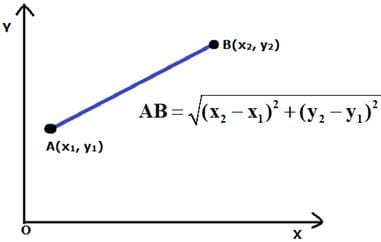XA = √{(2 – x)2 + (– 5 – 0)2}
= √{(2 – x)+ (– 5)2}
= √{4 + x2 – 4x + 25}
⇒ XA = √{29 + x2 – 4x}
XB = √{(– 2 – x)2 + (9 – 0)2}
= √{(– 2 – x)2 + (9)2}
= √{4 + x2 + 4x + 81}
⇒ XB = √{85 + x2 + 4x}
Now since
XA = XB
Squaring both sides, we get,
(29 + x2 – 4x) = (85 + x2 + 4x)
56 = – 8x
x = – 7
The point on × axis is (– 7, 0).

Q.16. Find points on the x – axis, each of which is at a distance of 10 units from the point A(11, – 8).

Let the point be X(x, 0)
XA = 10
By distance formula, as shown below: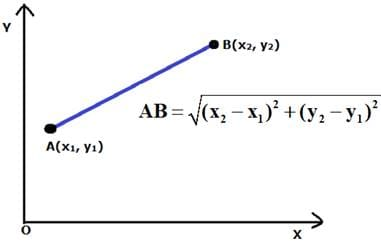XA = √{(11 – x)2 + (– 8 – 0)2}
10 = √{(11 – x)2 + (– 8)2}
10 = √{121 + x2 – 22x + 64}
10 = √{185 + x2 – 22x}
Squaring both sides we get
100 = (185 + x2 – 22x)
⇒ 85 + x2 – 22x = 0
⇒ x– 22x + 85 = 0
⇒ (x – 5)(x – 17)
⇒ × = 5 or × = 17
The points are (5, 0) and (17, 0).

Q.17. Find the point on the y – axis which is equidistant from the points A(6, 5) and B(– 4, 3).

Let the point be Y(0,y) and the other two points given as A(6,5) and B(– 4,3)
Given YA = YB
By distance formula, as shown below: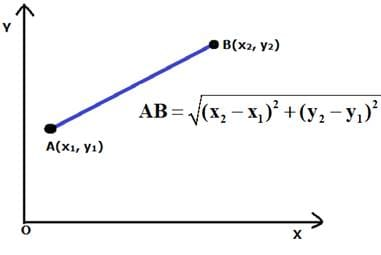YA = √{(6 – 0)2 + (5 – y)2}
= √{(6)2 + (5 – y)2}
= √{36 + 25 + y2 – 10y}
⇒ YA = √{61 + y2 – 10y}
YB = √{(– 4 – 0)2 + (3 – y)2}
= √{(– 4)+ (9 + y– 6y)}
= √{16 + 9 + y2 – 6y}
⇒ YB = √{25 + y2 – 6y}
Now, YA = YB
Squaring both sides, we get,
(61 + y2 – 10y) = (25 + y2 – 6y)
36 = 4y
⇒ y = 9
The point is (0, 9).

Q.18. If the point P(x, y) is equidistant from the points A(5, 1) and B(– 1, 5), prove that 3x = 2y.

The point P(x, y) is equidistant from the points A(5, 1) and B(– 1, 5), means PA = PB
By distance formula, as shown below: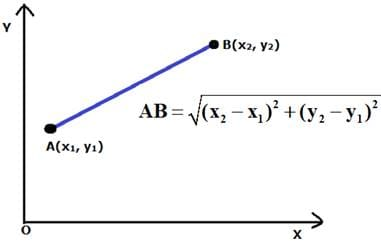PA = √{(5 – x)2 + (1 – y)2}
= √{(25 + x2 – 10x) + (1 + y2 – 2y)}
⇒ PA = √{26 + x2 – 10x + y2 – 2y}
PB = √{(– 1 – x)2 + (5 – y)2}
= √{(1 + x2 + 2x + 25 + y2 – 10y)}
⇒ PB = √{(26 + x2 + 2x + y2 – 10y)}
Now, PA = PB
Squaring both sides, we get
26 + x2 – 10x + y2 – 2y = 26 + x2 + 2x + y2 – 10y
⇒ 12x = 8y
⇒ 3x = 2y
Hence proved.

Q.19. If P(x, y) is a point equidistant from the points A(6, – 1) and B(2, 3), show that × – y = 3.

By distance formula, as shown below: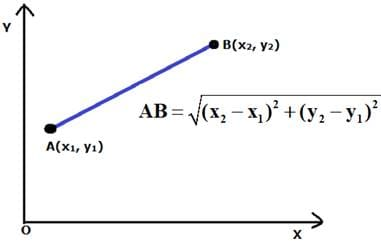PA = √{(6 – x)2 + (– 1 – y)2}
= √{(36 + x2 –12x) + (1 + y2 + 2y)}
⇒ PA = √{37 + x2 – 12x + y2 + 2y}
PB = √{(2 – x)2 + (3 – y)2}
= √{(4 + x2 – 4x + 9 + y2 – 6y)}
⇒ PB = √{(13 + x2 – 4x + y2 – 6y)}
Given: PA = PB
Squaring both sides, we get
(37 + x2 – 12x + y2 + 2y) = (13 + x2 – 4x + y2 – 6y)
24 = 8x – 8y
Dividing by 8
x – y = 3
Hence proved.

Q.20. Find the coordinates of the point equidistant from three given points A(5, 3), B(5, – 5) and C(1, – 5).

Let the point be P(x, y), then since all three points are equidistant therefore
PA = PB = PC
By distance formula, as shown below:We have, PA = √{(5 – x)2 + (3 – y)2}
= √{25 + x2 – 10x + 9 + y2 – 6y}
⇒ PA = √{34 + x2 – 10x + y2 – 6y}
PB = √{(5 – x)2 + (– 5 – y)2}
= √{25 + x2 – 10x + 25 + y2 + 10y}
⇒ PB = √{50 + x2 – 10x + y2 + 10y}
PC = √{(1 – x)2 + (– 5 – y)2}
= √{1 + x2 – 2x + 25 + y2 + 10y}
⇒ PC = √{26 + x2 – 2x + y2 + 10y}
Squaring PA and PB we get
{34 + x2 – 10x + y2 – 6y} = {50 + x2 – 10x + y2 + 10y}
⇒ – 16 = 16y
⇒ y = – 1
Squaring PB and PC we get
{50 + x2 – 2x + y+ 10y} = {26 + x2 – 10x + y2 + 10y}
24 = – 8x
x = – 3
P(– 3, – 1)

Q.21. If the points A(4, 3) and B(x, 5) lie on a circle with the centre O(2, 3), find the value of x.

OA = √{(4 – 2)2 + (3 – 3)2}
= √4
= 2
OB = √{(x – 2)2 + 4 }
= √{x2 + 4 – 4x + 4}
√{ 8 + x2 – 4x}
OA= OB2
4 = 8 + x2 – 4x
⇒ x2 – 4x + 4 = 0
⇒ x2 – 2x – 2x + 4 = 0
⇒ x(x– 2) – 2(x – 2) = 0
⇒ (x – 2) (x – 2) = 0
x = 2

Q.22. If the point C(– 2, 3) is equidistant from the points A(3, – 1) and B(x, 8), find the values of x. Also, find the distance BC.

By distance formulaAC = √{(3 – (– 2))2 + (– 1 – 3)2}
= √{(5)2 + (– 4)2}
= √{25 + 16}
= √{41}
BC = √{(x –(– 2))2 + (8 – 3)2}
= √{(x + 2)2 + 52 }
= √{x2 + 4 + 2x + 25}
= √{x+ 2x + 29}
AB = BC
√{x2 + 2x + 29} = √{41}
× = 2 or × = – 6
Since, AB = BC
BC = √41 units

Q.23. If the point P(2, 2) is equidistant from the points A(– 2, k) and B(– 2k, – 3), find k. Also, find the length of AP.

AP = BP
AP = √{(– 2 – 2)2 + (k – 2)2}
= √{16 + k2 – 4k + 4}
= √(k2 – 2k + 20)
BP = √{(– 2k – 2)+ (– 3 – 2)2}
= √{4k2 + 8k + 4 + 25}
= √(4k2 + 8k + 29)
Squaring AP and BP and equating them we get
k2 – 4k + 20 = 4k2 + 8k + 29
3k2 + 12k + 9 = 0
(k + 3)(k + 1) = 0
⇒ k = – 3
⇒ AP = √41units
Or k = – 1
⇒ AP = 5 units

Q.24. If the point (x, y) is equidistant from the points (a + b, b – a) and (a – b, a + b), prove that bx = ay.

Let point P(x,y) , A(a + b,a – b) , B(a – b,a + b)
Then AP = BP
AP = √{((a + b) – x)2 + ((a – b) – y)2}
= √{(a + b)2 + x2 – 2(a + b)x + (a – b)2 + y– 2(a – b)y}
= √(a2 + b2 + 2ab + x2 – 2(a + b)x + b+ a2 – 2ab + y2 – 2(a – b)y)
BP = √{((a – b) – x)+ ((a + b) – y)2}
= √{(a – b)2 + x2 – 2(a – b)x + (a + b)+ y2 – 2(a + b)y}
= √(a2 + b2 – 2ab + x2 – 2(a – b)x + b2 + a2 + 2ab + y2 – 2(a + b)y)
Squaring and Equating both we get
a2 + b2 + 2ab + x2 – 2(a + b)x + b2 + a2 – 2ab + y2 – 2(a – b)y = a2 + b– 2ab + x– 2(a – b)x + b2 + a2 + 2ab + y2 – 2(a + b)y
– 2(a + b)x – 2(a – b)y = – 2(a – b)x – 2(a + b)y
ax + bx + ay – by = ax – bx + ay + by
Hence
bx = ay

Q.25. Using the distance formula, show that the given points are collinear:
(i) (1, – 1), (5, 2) and (9, 5)
(ii) (6, 9), (0, 1) and (– 6, – 7)
(iii) (– 1, – 1), (2, 3) and (8, 11)
(iv) (– 2, 5), (0, 1) and (2, – 3).

Three or more points are collinear, if slope of any two pairs of points is same. With three points A, B and C if Slope of AB = slope of BC = slope of AC
then A, B and C are collinear points.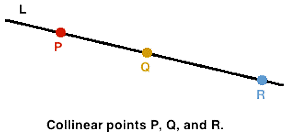Slope of any two points is given by:
(y2 – y1)/(x2 – x1).
(i) Slope of AB = (2 – (– 1))/(5 – 1) = 3/4
Slope of BC = (5 – 2)/(9 – 5) = 3/4
Slope of AB = slope of BC
Hence collinear.
(ii) Slope of AB = (1 – 9)/(0 – 6) = 8/6 = 4/3
Slope of BC = (– 6 – 0)/(– 7 – 1) = 6/6 = 1
Slope of AC = (– 7 – 9)/(– 6 – 6) = – 16/ – 12 = 4/3
Slope of AB = slope of AC
Hence collinear.
(iii) Slope of AB = ((3 – (– 1))/((2 – (– 1)) = 4/3
Slope of BC = (11 – 2)/(8 – 3) = 9/5 = 1
Slope of AC = ((11 – (– 1))/((8 – (– 1)) = 12/9 = 4/3
Slope of AB = slope of AC
Hence collinear.
(iv) Slope of AB = (1 – 5)/((0 – (– 2)) = – 4/2 = – 2
Slope of BC = (– 3 – 1)/(2 – 0) = – 4/2 = – 2
Slope of AB = slope of AB
Hence collinear.

Q.26. Show that the points A(7, 10), B(– 2, 5) and C(3, – 4) are the vertices of an isosceles right triangle.

In an isosceles triangle any two sides are equal.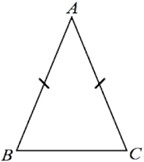AB = √{(– 2 – 7)2 + (5 – 10)2}

= √{(– 9)2 + (– 5)2}
= √{81 + 25}
= √{106}
BC = √{(– 4 – 5)2 + (3 – (– 2))2}
= √{(– 9)2 + (5)2}
= √{81 + 25}
= √{106}
AB = BC
∴ It is an isosceles triangle.

Q.27. Show that the points A(3, 0), B(6, 4) and C(– 1, 3) are the vertices of an isosceles right triangle.

In an isosceles triangle any two sides are equal.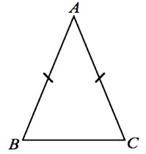AB = √{(6 – 3)2 + (4 – 0)2}
= √{(3)2 + (4)2}
= √{9 + 16}
= √{25} = 5 units
BC = √{(– 1 – 6)2 + (3 – 4)2}
= √{(– 7)2 + (– 1)2}
= √{49 + 1}
= √{50}
AC = √{(– 1 – 3)2 + (3 – 0)2}
= √{(– 4)2 + (3)2}
= √{16 + 9}
= √{25} = 5 units
AB = AC
∴ It is an isosceles triangle.

Q.28. If A(5, 2), B(2, – 2) and C(– 2, t) are the vertices of a right triangle with ∠B = 90°, then find the value of t.

A(5, 2), B(2, – 2) and C(– 2, t) are the vertices of a right triangle with ∠B = 90°
The value of t.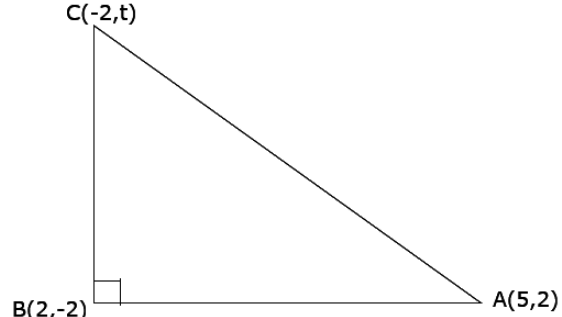From the fig we have ∠B = 90°,
so by Pythagoras theorem we have AC2 = AB2 + BC2
AC= (– 2 – 5)2 + (t – 2)2
= (– 7)2 + t2 + 4 – 2t
= 49 +t+ 4 - 2t
= 53 + t2 – 2t
AB2 = (2 – 5)+ (– 2 – 2)2
=(-3)2 + (–4)2
= 9 + 16
= 25
BC2 = (– 2 – 2)2 + (t + 2)2
= (– 4)2 + (t + 2)2
= 16 + t2 + 4 + 2t
= 20 + t2 + 2t
AB+ BC2 = 25 + 20 + t2 + 2t
= 45 + t2 + 2t
AC2 = 53 + t– 2t
⇒ 53 + t2 – 2t = 45 + t2 + 2t
⇒ 53 - 45 = 4t
⇒ 8 = 4t
⇒ t = 2

Q.29. Prove that the points A(2, 4), B(2, 6) and C(2 + √3, 5) are the vertices of an equilateral triangle.

For an equilateral triangle
AB = BC = AC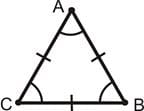AB = √{(6 – 4)2 + (2 – 2)2}
= √{(2)2 + 0}
= √{4 + 0}
= √{4} = 2 units
BC = √{(2 + √3 – 2)+ (5 – 6)2}
= √{3 + (– 1)2}
= √{4} = 2 units
AC = √{(2 + √3 – 2)2 + (5 – 4)2}
= √{3 + (– 1)2}
= √{4} = 2 units
Hence , AB = BC = AC
∴ ABC is an equilateral triangle.

Q.30. Show that the points (– 3, – 3), (3, 3) and (– 3√3, 3√3) are the vertices of an equilateral triangle.

Let the points be 3 (–3, –3), B (3, 3) and C (–3√3, 3√3)
Then, AB = √(3 + 3)2+( 3 + 3)2
=√(-6)2+(6)2
= √36+36
= √72
= 3√8
BC=√(-3√3+3)2+(3√3-3)2
= √(1-√3)232+(√3+1)232
= 3√[ 1+3-2√3+3+1+2√3]
= 3√8
CA = √(-3√3-3)2+(3√3-3)2
= √(-√3-1)232+(√3-1)232
= 3√[3+1+2√3+3+1-2√3]
= 3√8
∵ AB = BC = CA
⇒ A, B, C are the vertices of an equilateral triangle.

Q.31. Show that the points A(– 5, 6), B(3, 0) and C(9, 8) are the vertices of an isosceles right – angled triangle. Calculate its area.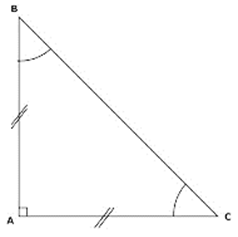AB = √{(0 – 6)2 + (3 – (– 5))2}
= √{(– 6)2 + (8)2}
= √{36 + 64}
= √{100} = 10 units
BC = √{(9 – 3)2 + (8 – 0)2}
= √{(6)2 + (8)2}
= √{36 + 64}
= √{100} = 10 units
AC = √{(9 – (– 5))2 + (8 – 6)2}
= √{(14)2 + (2)2}
= √{196 + 4}
= √{200}
For the right angled triangle
AC2 = AB+ BC2
AC2 = 200
AB2 + AC= 100 + 100 = 200
Since AB = BC
∴ ABC is an isosceles triangle.
Area = 1/2 (AB) (BC)
= 1/2 (10) (10)
= 1/2 (100)
= 50 sq units

Q.32. Show that the points 0(0, 0), A(3, √3) and B(3, – √3) are the vertices of an equilateral triangle. Find the area of this triangle.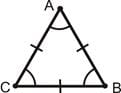OA = √{(√3)2 + (3 – 0)2}
= √{(3) + (3)2}
= √{3 + 9}
= √{12}
AB = √{(– √3 – √3)2 + (3 – 3)2}
= √{ – 2√3)2}
= √{12}
OB = √{(3 – 0)2 + (– √3 – 0)2}
= √{9 + 3}
= √{12}
Since OA = AB = OB , ∴ equilateral triangle.
Area = 1/2 [x1(y2 – y3) + x2(y3 – y1) + x3(y1 – y2)]
= 1/2[ – 3√3 – 3√3 ]
= – 3√3 sq units

Q.33. Show that the following points are the vertices of a square:
A(3, 2), B(0, 5), C(– 3, 2) and D(0, – 1)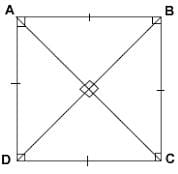AB = √{(0 – 3)2 + (5 – 2)2} = √{9 + 9} = √18 units
BC = √{(– 3 – 0)+ (2 – 5)2} = √{9 + 9} = √18 units
CD = √{(0 – (– 3))2 + (– 1 – 2)2} = √{9 + 9} = √18 units
DA = √{(0 – 3)2 + (– 1 – 2)2} = √{9 + 9} = √18 units
AC = √{(– 3 – 3)2} = √36 = 6 units
BD = √{(– 1 – 5)2} = √36 = 6 units
Since AB = BC = CD = DA and AC = BD
∴ ABCD is a square.

Q.34. Show that the following points are the vertices of a square:
A(6, 2), B(2, 1), C(1, 5) and D(5, 6)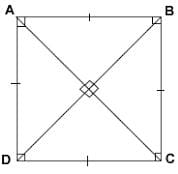AB = √{(2 – 6)2 + (1 – 2)2} = √{16 + 1} = √17 units
BC = √{(1 – 2)2 + (5 – 1)2} = √{1 + 16} = √17 units
CD = √{(5 – 1)2 + (6 – 5)2} = √{16 + 1} = √17 units
DA = √{(5 – 6)2 + (6 – 2)2} = √{16 + 1} = √17 units
AC = √{(1 – 6)2 + (5 – 2)2} = √{25 + 9} = √34 units
BD = √{(5 – 2)2 + (6 – 1)2} = √{25 + 9} = √34units
Since AB = BC = CD = DA and AC = BD
∴ ABCD is a square.

Q.35. Show that the following points are the vertices of a square:
A(0, – 2), B(3, 1), C(0, 4) and D(– 3, 1)AB = √{(3 – 0)2 + (1 – (– 2))2} = √{9 + 9} = √18 units
BC = √{(0 – 3)2 + (4 – 1)2} = √{9 + 9} = √18 units
CD = √{(– 3 – 0)+ (1 – 4)2} = √{9 + 9} = √18 units
DA = √{(– 3 – 0)2 + (1 – (– 2))2} = √{9 + 9} = √18 units
AC = √{ (4 – (– 2))2} = √{36} = 6 units
BD = √{(– 3 – 3)2 + (1 – 1)2} = √{36} = 6units
Since AB = BC = CD = DA and AC = BD
∴ ABCD is a square.

Q.36. Show that the points A(– 3, 2), B(– 5, – 5), C(2, – 3) and D(4, 4) are the vertices of a rhombus. Find the area of this rhombus. HINT Area of a rhombus = 1/2 × (product of its diagonals).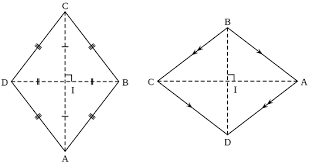AC = √{(2 – (– 3))2 + (– 32)2} = √{25 + 25} = √50 units
BD = √{(4 – (– 5))2 + (4 – (– 5))2} = √{81 + 81} = √162 units
Area = 1/2× (product of diagonals)
= 1/2 × √50 × √162
= 45 sq units

Q.37. Show that the points A(3, 0), B(4, 5), C(– 1, 4) and D(– 2, – 1) are the vertices of a rhombus. Find its area.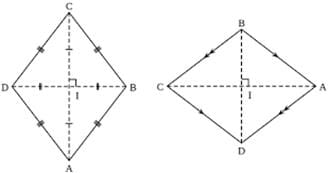AB = √{(4 – 3)2 + (5 – 0)2} = √{1 + 25} = √26 units
BC = √{(– 1 – 4)2 + (4 – 5)2} = √{25 + 1} = √26 units
CD = √{(– 2 – (– 1))2 + (– 1 – 4)2} = √{1 + 25} = √26 units
DA = √{(– 2 – 3)2 + (0 – 1)2} = √{25 + 1} = √26 units
AC = √{ (– 1 – 3)2 + (4 – 0)2} = √{32}
BD = √{(– 2 – 4)2 + (– 1 – 5)2} = √{36 + 36} = 6√2units
Since AB = BC = CD = DA
Hence, ABCD is a rhombus
Area = 1/2 × (product of diagonals)
= 1/2 × 4√2 × 6√2
= 24 sq units

Q.38. Show that the points A(6, 1), B(8, 2), C(9, 4) and D(7, 3) are the vertices of a rhombus. Find its area.AB = √{(8 – 6)2 + (2 – 1)2} = √{4 + 1} = √5 units
BC = √{(9 – 8)2 + (4 – 2)2} = √{1 + 4} = √5 units
CD = √{(7 – 9)2 + (3 – 4)2} = √{4 + 1} = √5 units
DA = √{(7 – 6)2 + (3 – 1)2} = √{1 + 4} = √5 units
AC = √{ (9 – 6)2 + (4 – 1)2} = √(9 + 9) = 3√2 units
BD = √{(7 – 8)2 + (3 – 2)2} = √{1 + 1} = √2 units
Since AB = BC = CD = DA
Hence, ABCD is a rhombus
Area = 1/2 × (product of diagonals)
= 1/2 × 3√2 × √2
= 3 sq units

Q.39. Show that the points A(2, 1), B(5, 2), C(6, 4) and D(3, 3) are the angular points of a parallelogram. Is this figure a rectangle?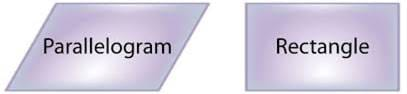AB = √{(5 – 2)2 + (2 – 1)2} = √{9 + 1} = √10 units
BC = √{(6 – 5)2 + (4 – 2)2} = √{1 + 4} = √5 units
CD = √{(3 – 6)2 + (3 – 4)2} = √{9 + 1} = √10 units
DA = √{(3 – 2)2 + (3 – 1)2} = √{1 + 4} = √5 units
Since AB = CD and BC = DA
∴ ABCD is Parallelogram
AC = √{(6 – 2)2 + (4 – 1)2} = √{16 + 9} = 5 units
For a Rectangle
AC2 = AB2 + BC2
Here AC2 = 25
But AB2 + BC2 = 15
∴ ABCD is not a rectangle

Q.40. Show that A(1, 2), B(4, 3), C(6, 6) and D(3, 5) are the vertices of a parallelogram. Show that ABCD is not a rectangle.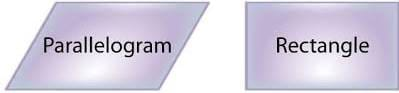AB = √{(4 – 1)2 + (3 – 2)2} = √{9 + 1} = √10 units
BC = √{(6 – 4)2 + (6 – 3)2} = √{4 + 9} = √13 units
CD = √{(6 – 3)2 + (5 – 6)2} = √{9 + 1} = √10 units
DA = √{(3 – 1)2 + (5 – 2)2} = √{4 + 9} = √13 units
AB = CD and BC = DA
∴ ABCD is a parallelogram
∴ AC = √{(6 – 1)2 + (6 – 2)2} = √{25 + 16} = √41 units
For a Rectangle
AC2 = AB2 + BC2
Here AC2 = 41
But AB+ BC2 = 23
∴ ABCD is not a rectangle

Q.41. Show that the following points are the vertices of a rectangle:
A(– 4, – 1), B(– 2, – 4), C(4, 0) and D(2, 3)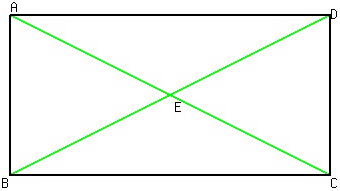A(– 4, – 1), B(– 2, – 4), C(4, 0) and D(2, 3)
AB = √{(– 2 – (– 4))2 + (– 4 – (– 1))2}
= √{4 + 9} = √13units
BC = √{(4 – (– 2))2 + (0 – (– 4))2}
= √{36 + 16} = √52units
CD = √{(2 – 4)2 + (3 – 0)2}
= √{4 + 9} = √13 units
DA = √{(2 – (– 4))2 + (3 – (– 1))2}
= √{36 + 16} = √52units
AB = CD and BC = DA
AC = √{(4 – (– 4))2 + (0 – (– 1))2}
= √{64 + 1} = √65 units
For a Rectangle
AC= AB2 + BC2
Here AC2 = 65
But AB+ BC= 13 + 52 = 65
∴ ABCD is a rectangle

Q.42. Show that the following points are the vertices of a rectangle:
A(2, – 2), B(14, 10), C(11, 13) and D(– 1, 1)AB = √{(14 – 2)2 + (10 – (– 2))2}
= √{144 + 144} = √288
BC = √{(11 – 14)+ (10 – 13)2}
= √{9 + 9} = √18 units
CD = √{(– 1 – 11)2 + (1 – 13)2}
= √{144 + 144}
= √288 units
DA = √{(– 1 – 2)+ (1 – (– 2))2}
= √{9 + 9} = √18units
AB = CD and BC = DA
AC = √{(11 – 2)2 + (13 – (– 2))2}
= √{81 + 225}
= √306 units
For a Rectangle
AC2 = AB2 + BC2
Here AC2 = 306
But AB+ BC2 = 288 + 18 = 306
∴ ABCD is a rectangle

Q.43. Show that the following points are the vertices of a rectangle:
A(0, – 4), B(6, 2), C(3, 5) and D(– 3, – 1)AB = √{(6 – 0)2 + (2 – (– 4))2}
= √{36 + 36}
= √72units
BC = √{(3 – 6)2 + (5 – 2)2}
= √{9 + 9}
= √18units
CD = √{(3 – (– 3))2 + (– 1 – 5)2}
= √{36 + 36}
= √72 units
DA = √{(– 3 – 0)2 + (– 1 – (– 4))2}
= √{9 + 9}
= √18units
AB = CD and BC = DA
AC = √{(3 – 0)2 + (5 – (– 4))2}
= √{9 + 81}
= √90 units
For a Rectangle
AC2 = AB2 + BC2
Here AC2 = 90
But AB2 + BC2 = 72 + 18 = 90
∴ ABCD is a rectangle

The document RS Aggarwal Solutions: Coordinate Geometry- 1 | RS Aggarwal Solutions for Class 10 Mathematics is a part of the Class 10 Course RS Aggarwal Solutions for Class 10 Mathematics.
All you need of Class 10 at this link: Class 10

53 docs|15 tests

## RS Aggarwal Solutions for Class 10 Mathematics

53 docs|15 testsExplore Courses for Class 10 examSignup to see your scores go up within 7 days! Learn & Practice with 1000+ FREE Notes, Videos & Tests.
10M+ students study on EduRev
Track your progress, build streaks, highlight & save important lessons and more!
Related Searches

,

,

,

,

,

,

,

,

,

,

,

,

,

,

,

,

,

,

,

,

,

;